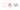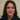# Using map method in React components with examples explained

It is interesting to know about using map method in React components. I came across it and wanted to explain it in a few examples in this post.

### Using map in Javascript

In a normal Javascript example this is how we use map method to create a new mapped array ( using arrow functions in practice):

``````const numbers = [1, 2, 3, 4, 5];
const doubled = numbers.map((number) => number * 2);``````

Obviously the numbers array is intact and we have created a new array which has doubled values for each key.

### Using map method in React example

To be able to render a list using  map method in React we could do the same thing:

``````const numbers = [1, 2, 3, 4, 5];
const listItems = numbers.map((number) =>
<li>{number}</li>
);``````

We just return the values in list items. And we can render it in our component in and unordered list:

``````ReactDOM.render(
<ul>{listItems}</ul>,
document.getElementById('root')
);``````

The only problem is although the list is showing but each child should have a unique key prop. A “key” is a special string attribute you need to include when creating lists of elements.

Using keys

Keys help React identify which items have changed, are added, or are removed. Keys should be given to the elements inside the array to give the elements a stable identity.

Using map method in React example with key

Therefor we add the key for each list item to make sure each element is unique and identifiable:

``````const numbers = [1, 2, 3, 4, 5];
const listItems = numbers.map((number) =>
<li key={number.toString()}>
{number}
</li>
);``````

So the above component will look like this using map method in React example:

``````function NumberList(props) {
const numbers = props.numbers;
const listItems = numbers.map((number) =>
<li key={number.toString()}>
{number}
</li>
);
return (
<ul>{listItems}</ul>
);
}

const numbers = [1, 2, 3, 4, 5];
ReactDOM.render(
<NumberList numbers={numbers} />,
document.getElementById('root')
);``````

Moreover we could inline the map results as we write JSX in React. So this is the same as above example:

``````function NumberList(props) {
const numbers = props.numbers;
return (
<ul>
{numbers.map((number) =>
<ListItem key={number.toString()}
value={number} />
)}
</ul>
);
}``````

A post example

As another example we can extract a post array with separate key value pairs using the id for the key:

``````const content = posts.map((post) =>
<Post
key={post.id}
id={post.id}
title={post.title} />
);``````

As a result we could extract whatever needed from the array as long as we specify the key for each item. Using map method in React makes it easy and convenient to pull out data out of the array like list items and use them in our components. Thanks for reading.